Next: 4.6 Dependence on the Up: 4.5 Kinematic arm model Previous: 4.5.1 Methods

## 4.5.2 Results

The abstract RNN could cope with the redundant arm postures for a given end-effector position; the MLP could not (table 4.3). The local PCA mixture approximated the training data also better then Neural Gas (table 4.3). The results from the different mixture models NGPCA, NGPCA-constV, and MPPCA-ext were almost equal (table 4.3). Compared to NGPCA, NGPCA-constV was slightly worse on the inverse direction. Over five different training cycles (retraining of the mixture of local PCA), the average position errors varied only slightly (for NGPCA, the maximum deviation was 2 mm).

Table 4.3: Position and collision errors for an abstract RNN using NGPCA, NGPCA-constV, and MPPCA-ext for training, compared to a variant using Neural Gas for training and to a multilayer perceptron (MLP). Results are shown for two different directions of recall: forward and inverse. The inverse model takes the desired collision state as an additional input variable (third column). Position errors are averaged over all test patterns, and are given with standard deviations. In the inverse case, the collision error is the percentage of trials deviating from the collision input value. In the forward case, it is the erroneous number of collision state predictions.

 method direction input position error (mm) collision error (%) NGPCA inverse no collision 27 ± 15 5 NGPCA inverse collision 23 ± 13 8 NGPCA forward - 44 ± 27 11 NGPCA-constV inverse no collision 31 ± 17 5 NGPCA-constV inverse collision 28 ± 14 11 NGPCA-constV forward - 43 ± 29 11 MPPCA-ext inverse no collision 29 ± 15 5 MPPCA-ext inverse collision 25 ± 14 6 MPPCA-ext forward - 45 ± 29 14 Neural Gas inverse no collision 58 ± 26 2 Neural Gas inverse collision 56 ± 27 4 Neural Gas forward - 160 ± 74 18 MLP inverse no collision 310 ± 111 30 MLP forward - 93 ± 48 13

The mixture models distribute the training patterns among the units of the mixture. For NGPCA and NGPCA-constV, every pattern is assigned to one unit (at the end of the training). The number of patterns assigned to a unit is a measure for the weight of the unit; for MPPCA-ext, the weights are the prior probabilities. These weights had roughly a bell-shaped distribution among the units (figure 4.10). Different from MPPCA-ext, the distributions for NGPCA and NGPCA-constV showed a second peak for units having few assigned patterns (around 50, the average is 250). A single peak seems to be favorable. However, the distribution of assigned patterns also depends on the structure of the data set (which is largely unknown). Apparently, in this experiment, the effect on the performance was negligible (table 4.3).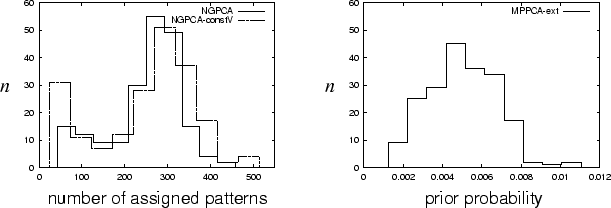The remaining tests were carried out only with NGPCA. The distribution of the individual errors shows regions corresponding to different ellipsoids selected during the recall (figure 4.11). At the transition between two regions, the error as a function of the input is discontinuous.

Figure: Position errors of the inverse model with input `collision'. (Left) Horizontal plane (approximately 70mm above the table). (Right) Vertical plane through the origin (z = 0).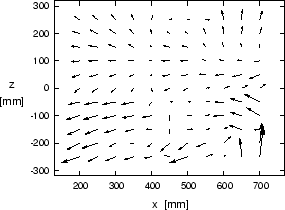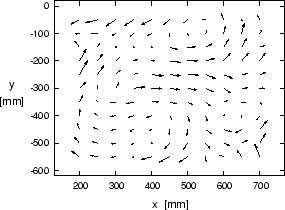The performance depends on the number of units m and principal components q. The position and the collision errors decreased with increasing m (table 4.4). Furthermore, the position error was smallest at q = 6 (figure 4.12, right). This q value matches the local dimensionality of the distribution (figure 4.12, left).

The abstract RNN could also cope with additional noise dimensions if the number of principal components was adjusted accordingly (table 4.5). With three noise dimensions and q = 6 principal components, the position errors of the abstract RNN were more than double . However, with q = 9, the position errors were again at the no-noise level.

Table 4.4: Dependence on the number m of units.

 direction input error m = 50 m = 100 m = 200 inverse no collision position (mm) 48 38 27 inverse no collision collision (%) 5 5 5 inverse collision position (mm) 47 35 23 inverse collision collision (%) 8 9 8 forward - position (mm) 74 56 44 forward - collision (%) 16 14 11

Figure 4.12: (Left) Ratio of successive averaged eigenvalues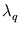and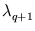(see methods). (Right) Dependence of the position error E (here for the direction: inverse, no collision) on the number of principal components q.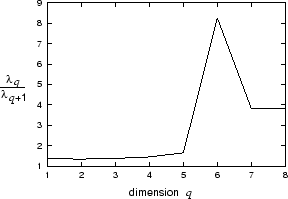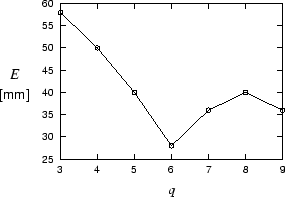Table 4.5: Compensation of noise. The first column of numbers shows the result without noise dimensions (as in table 4.3), the second with three noise dimensions and six principal components, and the third with noise and nine principal components.

 direction input error q = 6 (no noise) q = 6 q = 9 inverse no collision position (mm) 27 57 30 inverse no collision collision (%) 5 6 6 inverse collision position (mm) 23 64 24 inverse collision collision (%) 8 6 11 forward - position (mm) 44 101 45 forward - collision (%) 11 15 13

The errors for the forward direction were consistently higher than for the inverse direction (table 4.3 and 4.4). The major difference seems to be that the forward direction has six input dimensions; the inverse direction has only four. This is consistent with the finding that the square error per output dimension increased with the number of input dimensions (figure 4.13). For intermediate numbers r, the increase was even exponential. In the following section, this finding is investigated theoretically.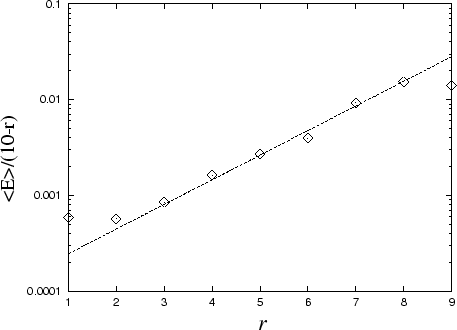Next: 4.6 Dependence on the Up: 4.5 Kinematic arm model Previous: 4.5.1 Methods
Heiko Hoffmann
2005-03-22